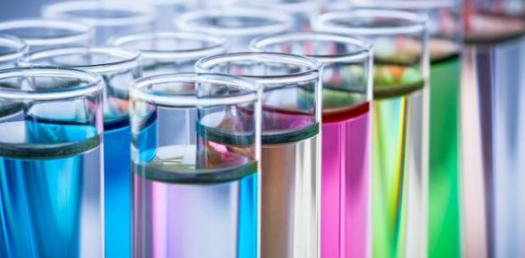# Hardest Trivia Questions On Chemistry For 11th Grade

10 Questions | Total Attempts: 133SettingsChemistry is study of matter:it's composition, properties, and reactivity. It is the science that involve elements and compound composed of atoms, molecules and ions. Many people think of chemist as being white coated scientists mixing strange liquids Ina laboratory but the truth is we are all chemist. Try out this quiz. Good luck!

• 1.
What is an atom?
• A.

Building blocks of protein

• B.

Building block of calcium

• C.

Building blocks of matter that join to form molecules

• D.

Building blocks of matter that join to form compound

• 2.
Which of the following is not part of the component of Atom?
• A.

Proton

• B.

Neuron

• C.

Electron

• D.

Ion

• 3.
Which one does proton carry?
• A.

Positive electric charge

• B.

Negative electric charge

• C.

Neutral charge

• D.

Semi positive charge

• 4.
What does electron Carry?
• A.

Positive electric charge

• B.

Negative electric charge

• C.

Neutral charge

• D.

Semi positive charge

• 5.
Which of the following can cause changes in atomic nucleus?
• A.

Physical reaction

• B.

Chemical reaction

• C.

Nuclear reaction

• D.

Fission reaction

• 6.
What determines the number of an element in the period?
• A.

Number of electron in its atom

• B.

Number of proton in it's atom

• C.

Number of neuron in its atom

• D.

Non of the above

• 7.
Which are cations?
• A.

Elements carrying positive charged ion

• B.

Metals

• C.

Elements carrying negative charged ion

• D.

Non of the above

• 8.
Which of the following is a cation?
• A.

O2-

• B.

He2+

• C.

Cl-

• D.

Fl-

• 9.
Which of the following is an anion?
• A.

He2+

• B.

Na+

• C.

K+

• D.

Cl-

• 10.
What determines the isotope of am element?
• A.

Number of electron

• B.

Number of proton

• C.

Number of neurons

• D.

Non of the above

Related TopicsBack to top